WEBINAR
Magnetic Levitation for Energy Harvesting
Thursday, June 22, 2023
Time
SESSION 1
SESSION 2
CEST (GMT +2)
03:00 PM
08:00 PM
EDT (GMT -4)
09:00 AM
02:00 PM
HOME / Applications / Calculation of thin film resistance using EMS

# Calculation of thin film resistance using EMS

Used Tools:## Thin film resistor

Thin film resistors are made of vacuum deposited homogeneous metallic thin film on an insulating substrate. They have smooth electron flow, small temperature coefficient of resistance, and are good fit for high precision application.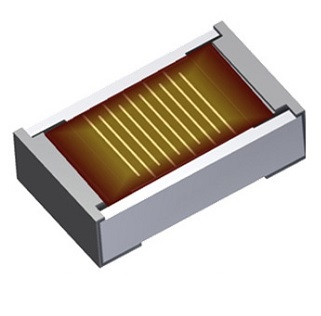Figure1 - Thin film resistance

## Thin film resistor model

In this example, EMS and SOLIDOWRKS are used to model and simulate a thin film resistor. Figures 2 and 3 show the geometrical parameters and the 3D model of the simulated resistor.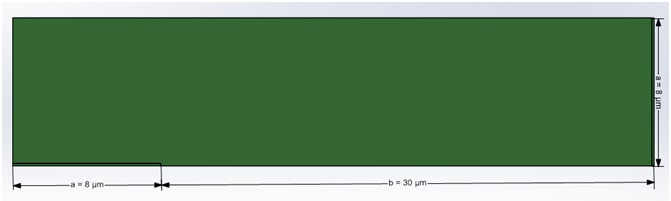Figure 2 - Model dimensions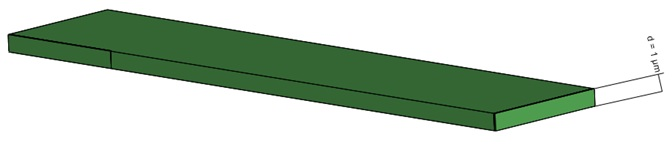Figure 3 - 3D Model of thin film resistor

## Electric Conduction simulation inside EMS

Electric Conduction module is primarily used for computing current flow in conductors due voltage differences. Results generated by this module are electrostatic potential, electrostatic field, current density, breakdown voltage, resistance. With capabilities of being coupled to thermal and motion analyses, Electric Conduction module helps to solve wide range of problems and covers several applications.

Electric Conduction Module is used in this example to compute resistance inside EMS. The resistance is defined between two points as the ratio of voltage and current. Therefore, the user has to specify the entry and exit ports for the resistor set. The entry port is where the current flows into the resistor and exit port is where the current exits. EMS automatically computes the voltage difference between the entry and exit ports as well as the current flowing through the resistor set.  From the current and voltage, the resistance is deduced.

## Simulation Setup

These four steps should be followed to perform an Electric Conduction simulation in EMS.

1. Create a new Electric Conduction study

2. Apply suitable materials to the parts

3. Apply suitable load and restraint

4. Mesh and Run the simulation

## Materials

In this case, the thin film resistor is made of metallic material with 2e+7 S/m conductivity.

Figure below shows the faces where the fixed voltages are applied. Their value are 0.001V in the left face and -0.001 in the right face. Resistance sets are applied also in the same faces.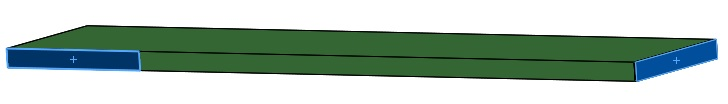Figure 4 - highlights of faces where fixed voltages and resistances are applied

## Mesh

Mesh is critical to all FEM simulation. The results accuracy and the solving time are strongly dependant on the mesh size. The models with the finer mesh (smaller element size) yield highly accurate results but will take longer computing time. Mesh can be generated automatically by EMS after estimating the global size of the model. Also, EMS offers extra meshing flexibility due to the possibility of controlling the mesh size on solid bodies and faces by the mesh control. In this example, the faces where fixed voltages are applied, are finely meshed, with the maximum element dimension of 0.1 um.  The whole model has maximum element size of 0.3 um.Figure 5 shows the final mesh.

## FEM results computed by EMS

After running the study. The above mentioned results are generated by EMS. To get more accurate results the option of Normal accuracy in the advanced study properties is changed to high accuracy. High accuracy option spends more solving time but gives more accurate results.

The resistance of the simulated thin film resistor can be calculated by the formula below :Figure 6 shows the resistance value computed by finite element method in EMS. Theoretical and EMS solution are almost identical. Figures 7 and 8 show respectively 3D plots of current density and electrostatic potential.Figure 6 - Resistance calculated by EMS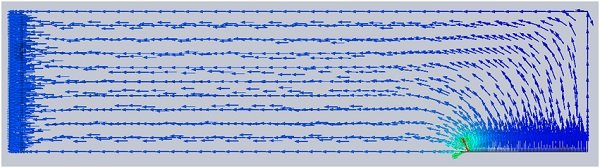Figure 7 - Current density, vector plot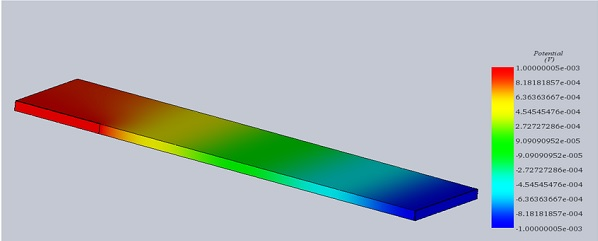Figure 8 - Potential plot

## Conclusion

Analytical results of a thin film resistance agree very well with the EMS.EMWorks’ low frequency package EMS, which is fully embedded inside a 3D CAD software (Solidworks, Autodesk inventor and SpaceClaim), helps engineers to optimize their productsand save time and money.

## References

: Conformal Mapping: Methods and Applications, Roland Schinzinger, Patricio A. A. Laura, 1991, Elsevier Science Publishers, B.V., p. 224. ISBN 0-486-43236-X (pbk).